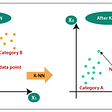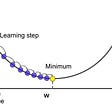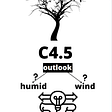# Table content:-

1. Definition
2. Types of Logistic Regression
3. Assumptions of Logistic Regression
4. Why Logistic Over Linear?
5. Odds Ratio and Logit
6. Logistic Regression Model
7. Cost function
8. Evaluation Metrics
9. References

# Different types of Logistic Regression are :-

1. Binomial Logistic Regression :- In this target variables can have only two possible outcome. ex :- Yes/No or Pass/fail etc.
2. Multinomial Logistic Regression :- In this target variables can have three or more possible outcome ( outcomes don’t have any quantitative significance ). ex :- diseases A, diseases B and diseases C etc.
3. Ordinal Logistic Regression :- It deals with the target variables with ordered categories. ex :-“poor”, “good”, “very good” etc.

# Assumptions of Logistic Regression :-

1. Binary logistic regression requires the dependent variables to be binary while the ordinal logistic regression requires the dependent variables to be ordinal.
2. Observations should not come from repeated measurements or matched data.
3. It requires no or little multicollinearity within independent variables which means that independent variables should not be too highly correlated with each other.
4. It assumes linearity of independent variables and log odds.
5. Logistic Regression typically requires a large sample size.

# Reason why linear Regression is not suitable for Classification problem :-

1. In Linear Regression predicted value is continuous, they are not probabilistic like Logistic Regression.
2. Linear regression is sensitive to outliers or imbalance data.
3. Linear regression can predict the probability from negative to positive infinity, but the probability can only lies between 0 and 1. To tackle such problem we use logit function or log-odds function.

# Evaluation of Logistic Regression Model

1. Akaike Information Criteria (AIC) :- AIC is the measure of fit which penalizes model for the number of model coefficients. Therefore, a model having minimum AIC value should always be considered.
2. Confusion Matrix :- Confusion matrix shows us the tabular representation of the actual and predicted value. Using this we can find the accuracy and it also helps us in avoiding overfitting.
3. Receiver Operating Characteristic ( ROC Curve ) :- Higher the area under curve, better the prediction power of the model.
1. Wikipedia
2. Analytics Vidhya Blogs
3. Few other sources

--

--

--

## More from Akash Patel

Love podcasts or audiobooks? Learn on the go with our new app.

## 5 Principles of Effective Data Visualization.## The 3 Essentials of Impactful Systems Analysis## SlicerJupyter: a 3D Slicer kernel for interactive publications## 5 Prime Benefits of Using Prescriptive Analytics by HData Systems## K — Nearest Neighbors Explained## How I Applied Machine Learning to Real Life for Planning My Trip to Hong Kong## More from Medium## Math in Logistic Regression!## Understanding C4.5 Decision tree algorithm## Linear Regression